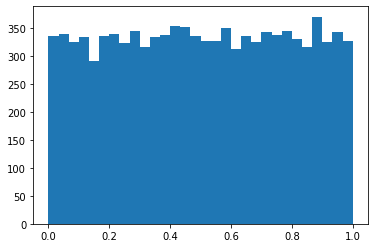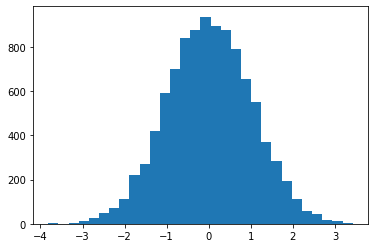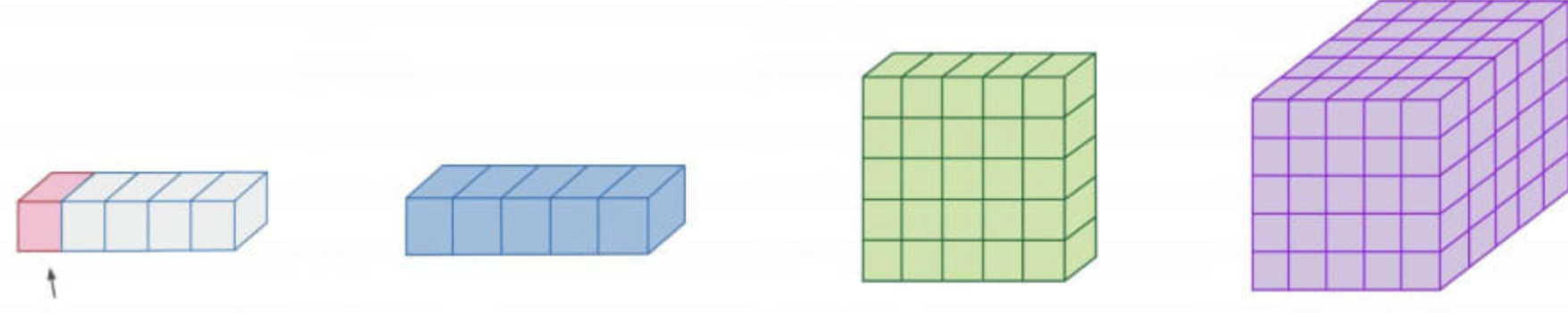# 數列運算¶

:

import numpy as np
import matplotlib.pyplot as plt


## 關於 NumPy¶

NumPy 是 Numerical Python 的縮寫，是 Python 使用者用來實踐向量化（Vectorization）、科學計算與資料科學的套件，也是我們進行「運算數值」的主角，資料科學團隊使用 NumPy 所提供一種稱為 ndarray 的資料結構來儲存並運算數值陣列、與標準套件 random 相輔相成的 numpy.random 來處理隨機性以及 numpy.linalg 來處理線性代數中一些繁複的運算。透過 ndarray 的數值陣列幾乎能夠面對任意的資料來源，包括實驗資料、圖像（表示像素亮度的二維數值陣列）或者音訊（表示強度與時間軸的一維數值陣列。） 熟悉 ndarray 的操作對於以 Python 應用資料科學的使用者來說是必要的前提，也扮演著理解機器學習理論的基石，如果能夠自信地操作二維數值陣列（即矩陣 Matrix）以及三維數值陣列（即張量 Tensor），將更容易理解高階機器學習框架究竟在封裝起來的函式、方法中提供了哪些功能給使用者，進而更有效率作超參數的調校。 我們可以在執行 Python 的載入指令（import）後印出 NumPy 的版本號來確認環境中是否安裝了 NumPy 可供使用。

:

print(np.__version__)

1.18.0


Traceback (most recent call last):
File "<stdin>", line 1, in <module>
ModuleNotFoundError: No module named 'numpy'


# 在命令列執行
pip install numpy


## 為何 NumPy¶

:

heterogeneous_list = [5566, 55.66, True, False, '5566']
for i in heterogeneous_list:
print(type(i))

<class 'int'>
<class 'float'>
<class 'bool'>
<class 'bool'>
<class 'str'>


list 中每個元素都是一個完整的 Python 物件，具備各自的類別與數值資訊，這使得它在計算同質資料時的效能、語法付出代價，我們需要透過迭代將裡面的物件一一取出後運算。

:

homogeneous_list = [1, 2, 3, 4, 5]
[i**2 for i in homogeneous_list]

:

[1, 4, 9, 16, 25]


NumPy 的 ndarraylist 的最大不同在於同質資料型態的特性，在計算時的效能和語法更快速與簡潔。

:

arr = np.array([1, 2, 3, 4, 5])
arr**2

:

array([ 1,  4,  9, 16, 25])


ndarray 在同質數值陣列計算的便利性相較原生 list 具有絕對的優勢，接著我們來暸解如何創建 ndarray 以及 NumPy 的標準資料型態。

## 如何創建 ndarray¶

:

homogeneous_list = [1, 2, 3, 4, 5]
type(homogeneous_list)

:

list

:

arr = np.array(homogeneous_list)
print(type(arr))
print(arr)
print(arr.dtype)

<class 'numpy.ndarray'>
[1 2 3 4 5]
int64


np.array() 可以搭配 dtype 參數指定資料型態，可以傳入下列常見的資料型態：

• int ：整數型態

• float ：浮點數型態

• bool ：布林型態

:

homogeneous_list = [1, 2, 3, 4, 5]
arr = np.array(homogeneous_list, dtype=int)
arr.dtype

:

dtype('int64')

:

arr = np.array(homogeneous_list, dtype=float)
arr.dtype

:

dtype('float64')


• np.zeros(shape)：創建指定外觀充滿 0 的數值陣列

• np.ones(shape) ：創建指定外觀充滿 1 的數值陣列

• np.full(shape, fill_value)：創建指定外觀充滿 fill_value 的數值陣列

:

np.zeros(5, dtype=int) # 外觀為 (5,)

:

array([0, 0, 0, 0, 0])

:

np.ones((2, 2), dtype=float) # 外觀為 (2, 2)

:

array([[1., 1.],
[1., 1.]])

:

np.full((2, 2), 5566, dtype=int) # 外觀為 (2, 2)

:

array([[5566, 5566],
[5566, 5566]])


• np.arange(start, stop, step)：創建從 start（包含）間隔 stepstop（不包含）的等差數列，使用方式同內建函式 range()

• np.linspace(start, stop, num) ：創建從 start（包含）至 stop（包含）的均勻切割為 num 個資料點的數值陣列

:

np.arange(1, 10, 2)

:

array([1, 3, 5, 7, 9])

:

np.linspace(1, 9, 5, dtype=int)

:

array([1, 3, 5, 7, 9])


• np.random.random(size)：創建指定外觀介於 0, 1 之間、並符合均勻分佈的數值陣列

• np.random.normal(loc, scale, size)：創建指定外觀以 loc 為平均數、scale 為標準差常態分佈的數值陣列

• np.random.randint(low, high, size)：創建指定外觀於 low （包含）到 high（不包含）之間隨機抽樣之正整數的數值陣列

:

uniform_arr = np.random.random(10000)
normal_arr = np.random.normal(0, 1, 10000)
randint_arr = np.random.randint(1, 7, size=6)
print(uniform_arr)
print(normal_arr)
print(randint_arr)

[0.07888432 0.85680097 0.59827821 ... 0.57373442 0.51850271 0.92199587]
[ 1.2202809  -0.42494108  0.09156555 ... -0.40549757  1.43683747
-1.45461075]
[1 6 5 5 4 6]


:

fig = plt.figure()
ax = plt.axes()
ax.hist(uniform_arr, bins=30)
plt.show():

fig = plt.figure()
ax = plt.axes()
ax.hist(normal_arr, bins=30)
plt.show()## 常用的 ndarray 屬性¶

ndarray 較常被用到的屬性有：

• arr.ndim：檢視 arr 有幾個維度

• arr.shape：檢視 arr 的外型

• arr.size：檢視 arr 的資料筆數，對一維陣列的意涵就像內建函式 len() 作用在 list 上一般

• arr.dtype ：檢視 arr 中同質資料的型態

:

arr = np.array([5, 5, 6, 6])
print(arr.ndim)
print(arr.shape)
print(arr.size)
print(arr.dtype)

1
(4,)
4
int64


## 純量、向量、矩陣與張量¶

• 純量：泛指沒有維度的數值

• 向量：泛指具有一個維度的數值陣列，我常喜歡用「吐司條」來比喻讓它更具體些

• 矩陣：泛指具有兩個維度的數值陣列，我常喜歡用「一片吐司」來比喻讓它更具體些

• 張量：泛指三個維度以及超過三個維度的數值陣列，我常喜歡用「多片吐司」來比喻讓它更具體些:

scalar = np.array(5566)
print(scalar)
print(scalar.ndim)
print(scalar.shape)

5566
0
()

:

# 吐司條
vector = np.array([5, 5, 6, 6])
print(vector)
print(vector.ndim)
print(vector.shape)

[5 5 6 6]
1
(4,)

:

# 一片 2x2 的吐司
matrix = np.array([5, 5, 6, 6]).reshape(2, 2)
print(matrix)
print(matrix.ndim)
print(matrix.shape)

[[5 5]
[6 6]]
2
(2, 2)

:

# 三片 2x2 的吐司
tensor = np.array([5, 5, 6, 6]*3).reshape(3, 2, 2)
print(tensor)
print(tensor.ndim)
print(tensor.shape)

[[[5 5]
[6 6]]

[[5 5]
[6 6]]

[[5 5]
[6 6]]]
3
(3, 2, 2)


NumPy 有非常豐富的內建函式可以協助我們進行常用的向量與矩陣計算。運用 np.eye() 可以創建單位矩陣（Identity matrix），函式命名是取 I 的諧音。

:

I = np.eye(matrix.shape, dtype=int)
I

:

array([[1, 0],
[0, 1]])


:

np.dot(vector, vector)

:

122

:

print(np.dot(matrix, I))
print(np.dot(I, matrix))

[[5 5]
[6 6]]
[[5 5]
[6 6]]

:

np.dot(matrix, matrix)

:

array([[55, 55],
[66, 66]])


:

matrix = np.arange(6).reshape(2, 3)
print(matrix)
print(np.transpose(matrix))
print(matrix.T)

[[0 1 2]
[3 4 5]]
[[0 3]
[1 4]
[2 5]]
[[0 3]
[1 4]
[2 5]]


\begin{align} ax &= b \\ \frac{1}{a}ax &= \frac{1}{a}b\\ x &= \frac{b}{a} \end{align}

\begin{align} AX &= B \\ A^{-1}AX &= A^{-1}B \\ X &= A^{-1}B \end{align}

:

A = np.array([1, 2, 3, 4]).reshape(2, 2)
B = np.array([5, 6, 7, 8]).reshape(2, 2)
A_inv = np.linalg.inv(A)
X = np.dot(A_inv, B)
X

:

array([[-3., -4.],
[ 4.,  5.]])


## ndarray 的索引¶

ndarray 中取出單個資料值的方式與 list 相同，使用 [INDEX] 取值，索引值同樣由左至右從 0 算起，由右至左從 -1 算起，在參數命名上稱呼最左邊為起始（start）、最右邊為終止（stop）。

:

arr = np.array([55, 66, 56, 5566])
print("From start to stop:")
print(arr)
print(arr)
print(arr)
print(arr[arr.size - 1])
print("From stop to start:")
print(arr[-1])
print(arr[-2])
print(arr[-3])
print(arr[-arr.size])

From start to stop:
55
66
56
5566
From stop to start:
5566
56
66
55


:

np.random.seed(42)
arr = np.random.randint(1, 10, size=(3, 4))
print(arr)
print(arr[1, 1])  # 3 located at (1, 1)
print(arr[2, -3]) # 4 located at (2, -3)

[[7 4 8 5]
[7 3 7 8]
[5 4 8 8]]
3
4


## ndarray 的切割¶

ndarray 中取出多個資料值的方式與 list 相同，使用 [start:stop:step] 取出陣列的片段，如果沒有指定 start 預設值 0、 stop 預設值 arr.size 意即最右邊、 step 預設值 1；有趣的設定是當 step 為 -1 時候的效果為反轉數列。

:

arr = np.arange(10, 20)
print(arr[::])   # 三個參數都預設值
print(arr[::2])  # step=2
print(arr[:5])   # stop=5, 不包含
print(arr[5:])   # start=5, 包含
print(arr[::-1]) # step=-1, 反轉

[10 11 12 13 14 15 16 17 18 19]
[10 12 14 16 18]
[10 11 12 13 14]
[15 16 17 18 19]
[19 18 17 16 15 14 13 12 11 10]


## ndarray 特別的索引¶

• 華麗索引（Fancy Indexing）

• 布林索引（Boolean Indexing）

:

np.random.seed(0)
arr = np.random.randint(1, 100, size=(10,))
odd_indices = [0, 2, 8]
print(arr)
print(arr[odd_indices])

[45 48 65 68 68 10 84 22 37 88]
[45 65 37]


:

is_odd = [True, False, True, False, False, False, False, False, True, False]
print(arr)
print(arr[is_odd])

[45 48 65 68 68 10 84 22 37 88]
[45 65 37]


:

is_odd = arr % 2 == 1
print(is_odd)
print(arr)
print(arr[is_odd])

[ True False  True False False False False False  True False]
[45 48 65 68 68 10 84 22 37 88]
[45 65 37]


## 重塑外觀¶

• reshape(m, n, ...)

• ravel()

:

arr = np.arange(1, 10)
print(arr)
print(arr.shape)
print(arr.reshape(3, 3))
print(arr.reshape(3, 3).shape)

[1 2 3 4 5 6 7 8 9]
(9,)
[[1 2 3]
[4 5 6]
[7 8 9]]
(3, 3)


:

arr = np.arange(1, 10)
print(arr.reshape(3, -1))
print(arr.reshape(-1, 3))

[[1 2 3]
[4 5 6]
[7 8 9]]
[[1 2 3]
[4 5 6]
[7 8 9]]


:

arr = np.arange(1, 4)
print(arr.reshape(-1, 1)) # 重塑成二維的欄
print(arr.reshape(1, -1)) # 重塑成二維的列

[

]
[[1 2 3]]


:

arr = np.arange(1, 10).reshape(3, 3)
print(arr.shape)
print(arr.ndim)
print(arr.ravel().shape)
print(arr.ravel().ndim)

(3, 3)
2
(9,)
1


## 複製陣列¶

ndarray 有一個重要的預設特性為「不複製」，不論在切割或重新宣告的情境中都是創建陣列的 View，而非複製另一個陣列，這代表著對以 View 存在的子陣列（Sub-array）更新會改動到原始陣列。例如將 arr 的 View mat 之中的 5 更新為 5566，會一併更新 arr 的 5 為 5566。

:

arr = np.arange(1, 10)
mat = arr.reshape(3, 3)
mat[1, 1] = 5566
print(mat)
print(arr)

[[   1    2    3]
[   4 5566    6]
[   7    8    9]]
[   1    2    3    4 5566    6    7    8    9]


:

arr = np.arange(1, 10)
mat = arr.copy()
mat = mat.reshape(3, 3)
mat[1, 1] = 5566
print(mat)
print(arr)

[[   1    2    3]
[   4 5566    6]
[   7    8    9]]
[1 2 3 4 5 6 7 8 9]


## 合併陣列¶

:

arr_a = np.arange(1, 5).reshape(2, 2)
arr_b = np.arange(5, 9).reshape(2, 2)
print(np.concatenate([arr_a, arr_b]))         # 預設 axis=0
print(np.concatenate([arr_a, arr_b], axis=1)) # axis=1

[[1 2]
[3 4]
[5 6]
[7 8]]
[[1 2 5 6]
[3 4 7 8]]


## 通用函式¶

NumPy 之所以被譽為 Python 資料科學應用的基石，就是因為能透過通用函式（Universal function）實踐其他科學計算語言內建的向量化（Vectorization）功能，通用函式的作用是針對數值陣列中每個數值進行相同的運算，效率會高於使用迭代語法。我們可以在 Jupyter Notebook 中使用 %timeit 得知若想以迭代對一百萬筆隨機整數進行「倒數」的運算要花多少時間。

:

long_arr = np.random.randint(1, 101, size=1000000)
%timeit [1/i for i in long_arr]

416 ms ± 49.5 ms per loop (mean ± std. dev. of 7 runs, 1 loop each)


:

long_arr = np.random.randint(1, 101, size=1000000)
%timeit np.divide(1, long_arr)

1.61 ms ± 256 µs per loop (mean ± std. dev. of 7 runs, 1000 loops each)


• np.add()：同 + 運算符

• np.subtract()：同 - 運算符

• np.multiply()：同 * 運算符

• np.divide()：同 / 運算符

• np.power()：同 ** 運算符

• np.floor_divide()：同 // 運算符

• np.mod()：同 % 運算符

:

# 以 np.power 作為一個簡單示範
arr = np.arange(9)
print(arr)
print(arr**2)
print(np.power(arr, 2))

[0 1 2 3 4 5 6 7 8]
[ 0  1  4  9 16 25 36 49 64]
[ 0  1  4  9 16 25 36 49 64]


:

def is_prime(x):
div_cnt = 0
for i in range(1, x+1):
if x % i == 0:
div_cnt += 1
if div_cnt > 2:
break
return div_cnt == 2

is_prime_ufunc = np.vectorize(is_prime)
arr = np.arange(1, 12)
print("是否為質數：")
print(is_prime_ufunc(arr))

是否為質數：
[False  True  True False  True False  True False False False  True]


## 聚合函式¶

1. 能沿指定維度聚合

2. 多數具有可運算遺漏值的相對應函式

「能沿指定維度聚合」的特性意指在面對具有多個維度的數值陣列時，可以指定應用在某一個維度，舉例來說面對有兩個維度 (m, n) 的矩陣時可以單純應用 np.sum() 將整個矩陣中的數值加總，將得到一個輸出；如果指定 axis=0 則是將矩陣中的每欄（column）分別加總，將得到 n 個輸出、指定 axis=1 則是將矩陣中的每列（row）分別加總，將得到 m 個輸出。

:

mat = np.arange(1, 16).reshape(3, 5)
print(np.sum(mat))         # 1 個輸出
print(np.sum(mat, axis=0)) # 5 個輸出
print(np.sum(mat, axis=1)) # 3 個輸出

120
[18 21 24 27 30]
[15 40 65]


「多數具有可運算遺漏值的相對應函式」的特性意指在面對具有 np.NaN 遺漏值的陣列時，原型聚合函式會回傳 np.NaN，這時可以採用相對應之可計算遺漏值函式運算，將會忽略 np.NaN ，傳回其餘數值的摘要。

:

arr = np.arange(1, 16, dtype=float)
arr[-1] = np.NaN
print(arr)
print(np.sum(arr))
print(np.nansum(arr))

[ 1.  2.  3.  4.  5.  6.  7.  8.  9. 10. 11. 12. 13. 14. nan]
nan
105.0


• np.sum()np.nansum()

• np.prod()np.nanprod()

• np.mean()np.nanmean()

• np.median()np.nanmedian()

• np.std()np.nanstd()

• np.var()np.nanvar()

• np.min()np.nanmin()

• np.max()np.nanmax()

• np.argmin()np.nanargmin()

• np.argmax()np.nanargmax()

:

np.random.seed(42)
arr_0 = np.random.random((10, 1))
arr_1 = 1 - arr_0
arr = np.concatenate([arr_0, arr_1], axis=1)
arr

:

array([[0.37454012, 0.62545988],
[0.95071431, 0.04928569],
[0.73199394, 0.26800606],
[0.59865848, 0.40134152],
[0.15601864, 0.84398136],
[0.15599452, 0.84400548],
[0.05808361, 0.94191639],
[0.86617615, 0.13382385],
[0.60111501, 0.39888499],
[0.70807258, 0.29192742]])

:

np.argmax(arr, axis=0) # 矩陣中的每欄最大值所處的列數

:

array([1, 6])

:

np.argmax(arr, axis=1) # 矩陣中的每列最大值所處的欄數

:

array([1, 0, 0, 0, 1, 1, 1, 0, 0, 0])


## 延伸閱讀¶

1. NumPy: Learn (https://numpy.org/learn/)

2. Introduction to NumPy. In: Jake VanderPlas, Python Data Science Handbook (https://jakevdp.github.io/PythonDataScienceHandbook/02.00-introduction-to-numpy.html)